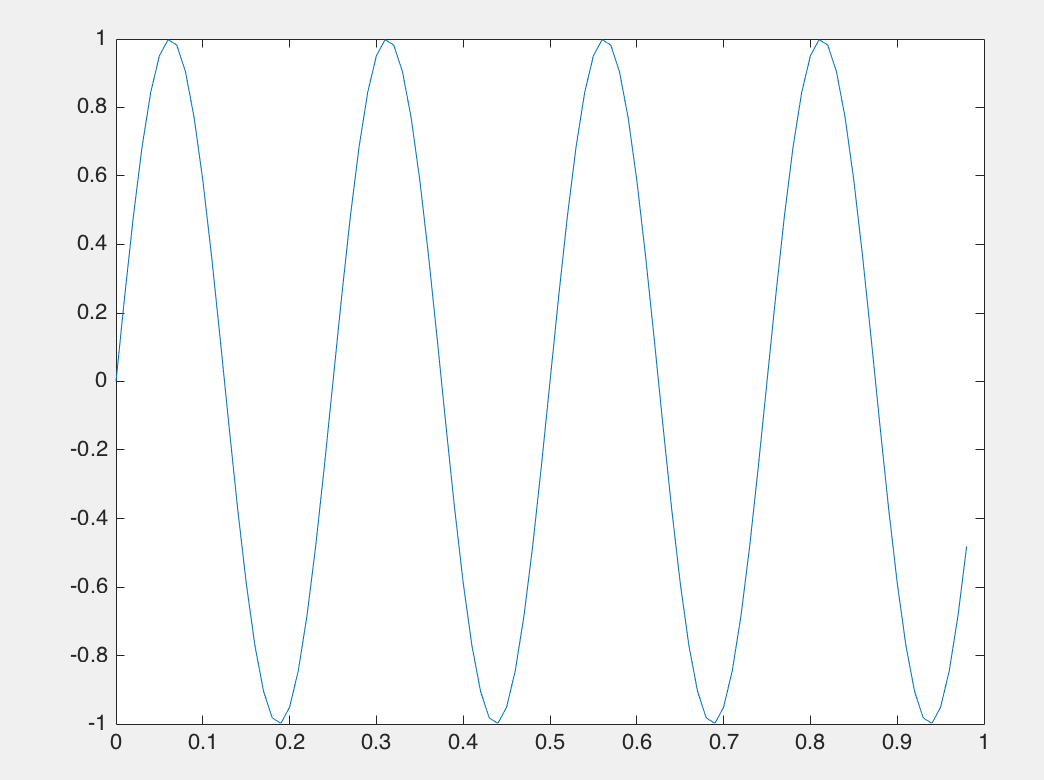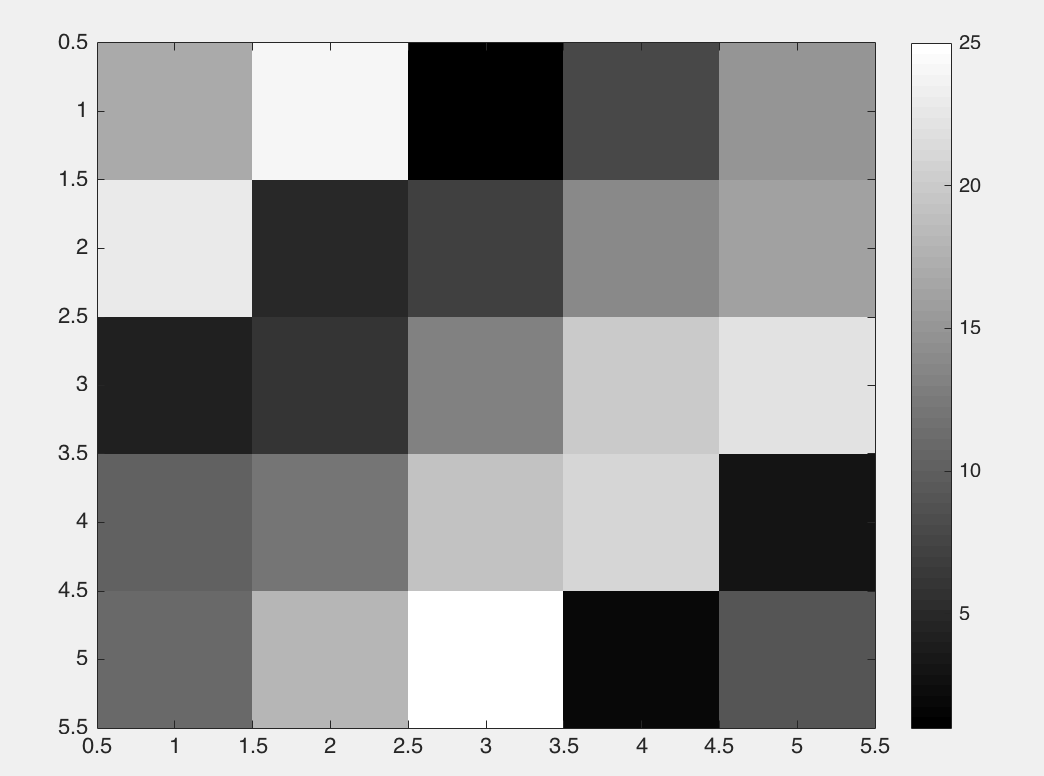# Octave与Matlab

Posted by jjx on December 1, 2016

Octave是matlab的开源免费版本，是matlab的有效替代工具，与matlab语法基本一致。

floor(a) 是向下四舍五入,因此对于 a 中的元素 0.5 将被下舍入变成 0。

t = (0:0.01:0.98);
y1 = sin(2*pi*4*t);
plot(t,y1);imagesc(A),colorbar,colormap gray